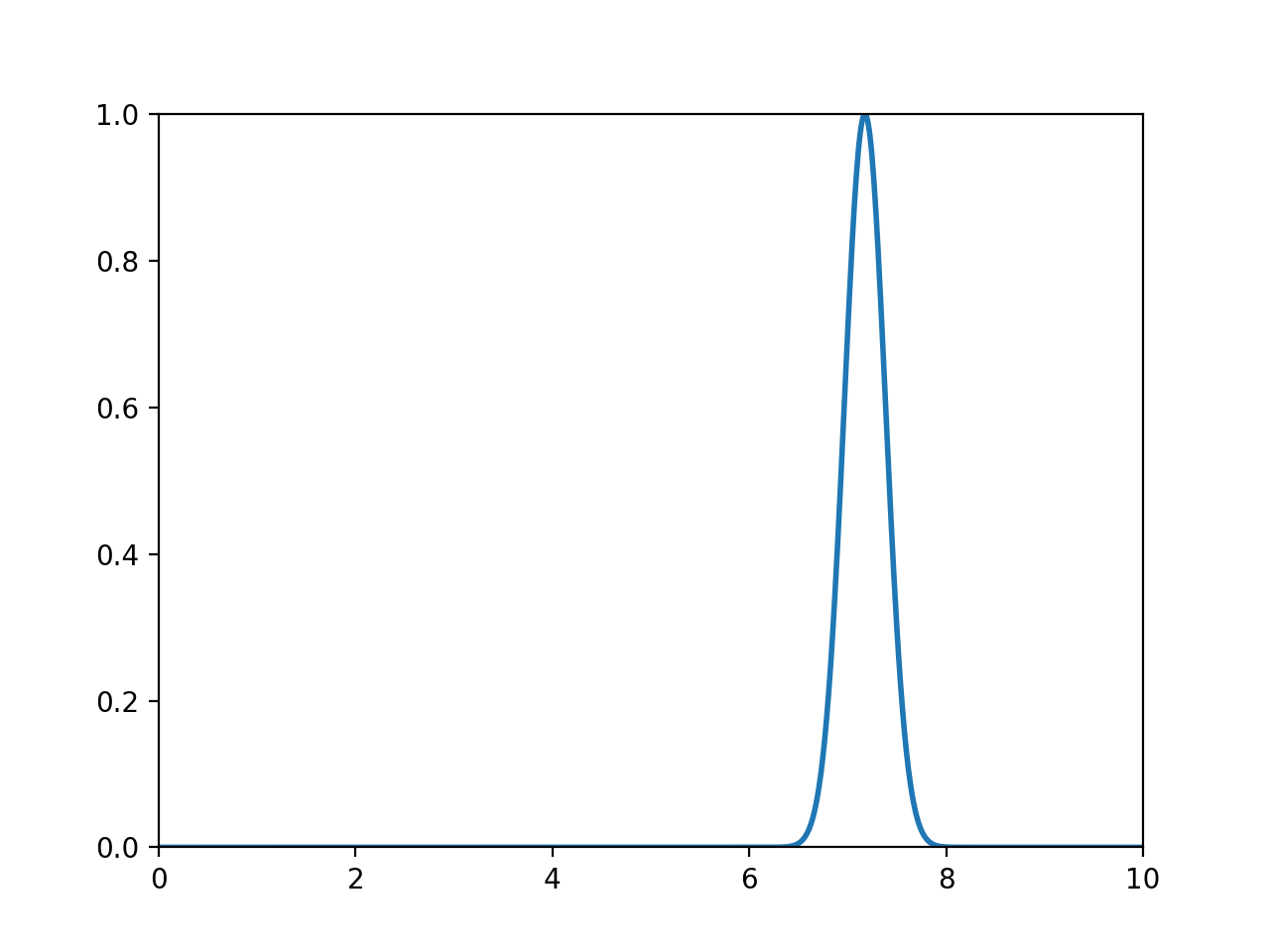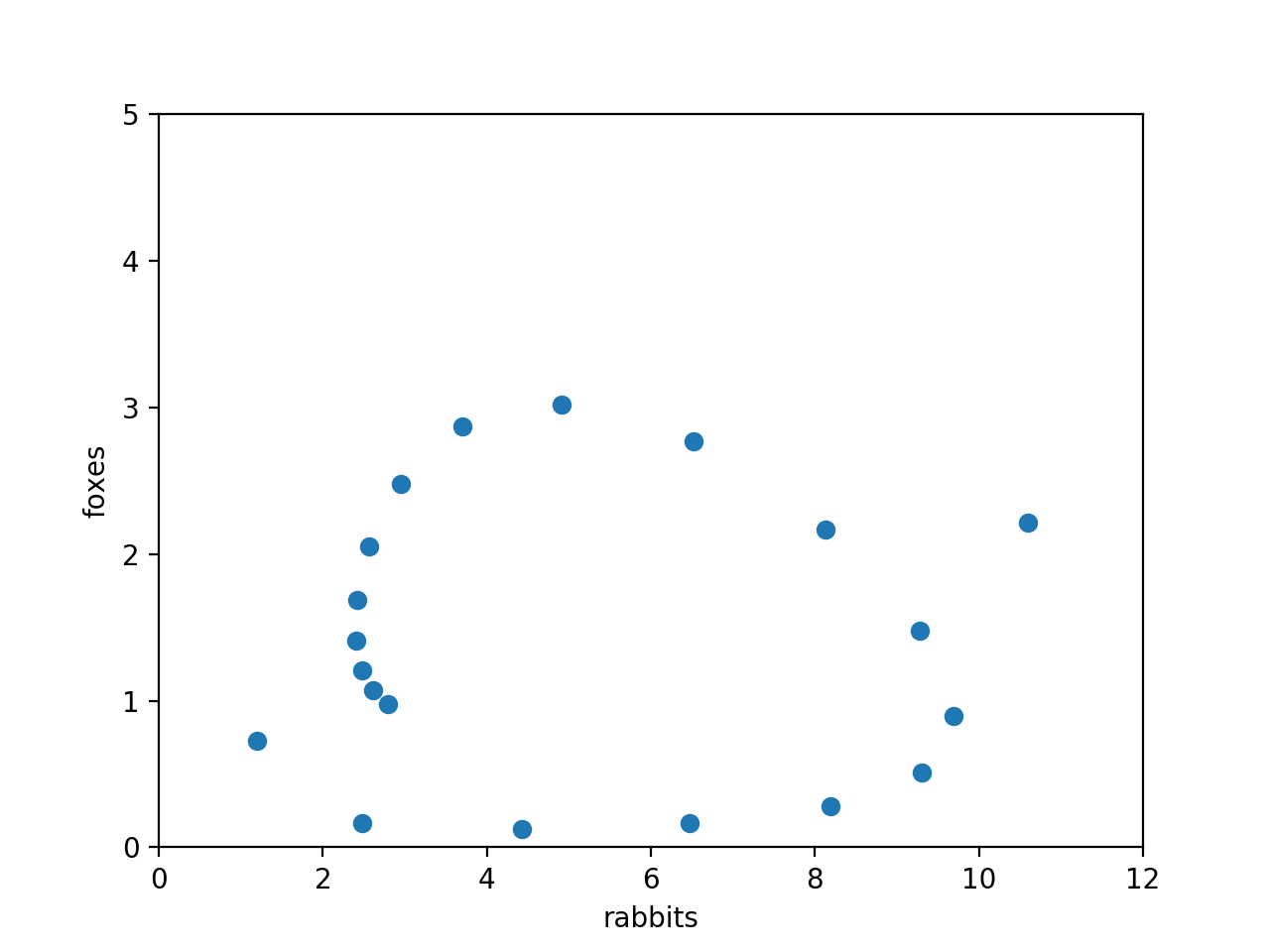In :
import numpy as np
import matplotlib.pyplot as plt
from matplotlib.animation import FuncAnimation
%matplotlib notebook


1. Testing the animations.

The codes to try are available on the course webpage.

2. Simple example

Below is modified code based on sinwave.py.

In :
fig = plt.figure()
ax = plt.axes(xlim=(0, 10), ylim=(0, 1))  # change the plot limits here
line, = ax.plot([], [], lw=2)

def init():  # Initialize with a blank plot
line.set_data([], [])
return line,

def animate(i):  # Plot a moving pulse
xs = np.linspace(0, 10, 1000)  # change the x range
x0 = 5*(1+np.sin(0.01*i))
x1 = 0.05*i
ys = np.exp(-10*(xs-x0)**2 / (1.5 + np.cos(x1)))
line.set_data(xs, ys)
return line,

anim = FuncAnimation(fig, animate, init_func=init,
frames=400, interval=20, blit=True)
# increase the number of frames to run for longer.3. Animating ODEs

Warning: There was a mistake in the equation for $\frac{dx}{dt}$ in the old version of the Lab Class sheet. The equations should read: \begin{eqnarray} \frac{dx}{dt} &=& x (1-gx) - \frac{xy}{1+hx} , \ \frac{dy}{dt} &=& -fy + \frac{xy}{1+hx} . \end{eqnarray}

Here I have modified the code in vanderpol.py

In :
from scipy.integrate import odeint

def dZ_dt(Z, t, f=8/3, g=3/50, h=3/20):
# Z is an array with two components
x, y = Z, Z  # rabbits and foxes
dxdt = x*(1-g*x) - x*y/(1+h*x)
dydt = -f*y + x*y/(1+h*x)
return [dxdt, dydt]

nlines = 20
rs = np.linspace(0.1, 3.0, nlines)  # the initial condition values
ts = np.linspace(0.0, 40.0, 1000)
linedata = []
for r in rs:
ic = (r, 1.0)  # the initial condition
linedata.append( odeint(dZ_dt, ic, ts) )

fig = plt.figure()
ax = plt.axes(xlim=(0,12), ylim=(0, 5), xlabel="rabbits", ylabel="foxes")
line, = ax.plot([], [], 'o')
npts = len(linedata[:,0])

def init():
line.set_data([],[])
return line,

def animate(i):
line.set_data([l[i,0] for l in linedata], [l[i,1] for l in linedata])
return line,

anim = FuncAnimation(fig, animate, init_func=init,
frames=npts, interval=50, blit=True)
# if you wait long enough, you will see evidence for a limit cycle here.In [ ]: